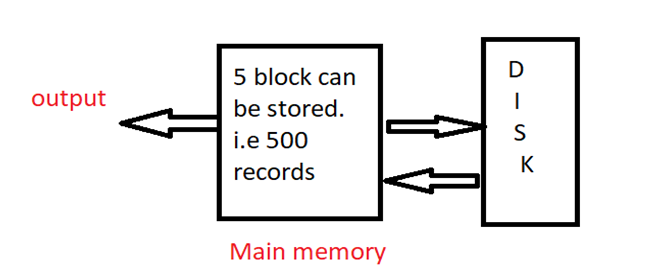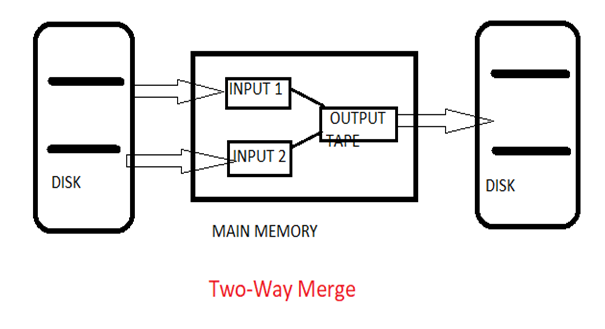# External Merge Sorting Algorithm

In this article, we will learn about the basic concept of external merge sorting. Example of external merge sorting with their algorithm.
Submitted by Abhishek Kataria, on June 29, 2018

## External sorting

External sorting is a technique in which the data is stored on the secondary memory, in which part by part data is loaded into the main memory and then sorting can be done over there. Then this sorted data will be stored in the intermediate files. Finally, these files will be merged to get a sorted data. Thus by using the external sorting technique, a huge amount of data can be sorted easily. In case of external sorting, all the data cannot be accommodated on the single memory, in this case, some amount of memory needs to be kept on a memory such as hard disk, compact disk and so on.

The requirement of external sorting is there, where the data we have to store in the main memory does not fit into it. Basically, it consists of two phases that are:

1. Sorting phase: This is a phase in which a large amount of data is sorted in an intermediate file.
2. Merge phase: In this phase, the sorted files are combined into a single larger file.

One of the best examples of external sorting is external merge sort.

## External merge sort

The external merge sort is a technique in which the data is stored in intermediate files and then each intermediate files are sorted independently and then combined or merged to get a sorted data.

For example: Let us consider there are 10,000 records which have to be sorted. For this, we need to apply the external merge sort method. Suppose the main memory has a capacity to store 500 records in a block, with having each block size of 100 records.In this example, we can see 5 blocks will be sorted in intermediate files. This process will be repeated 20 times to get all the records. Then by this, we start merging a pair of intermediate files in the main memory to get a sorted output.

### Two-Way Merge Sort

Two-way merge sort is a technique which works in two stages which are as follows here:

Stage 1: Firstly break the records into the blocks and then sort the individual record with the help of two input tapes.

Stage 2: In this merge the sorted blocks and then create a single sorted file with the help of two output tapes.

By this, it can be said that two-way merge sort uses the two input tapes and two output tapes for sorting the data.

### Algorithm for Two-Way Merge Sort:

Step 1) Divide the elements into the blocks of size M. Sort each block and then write on disk.

Step 2) Merge two runs

1. Read first value on every two runs.
2. Then compare it and sort it.
3. Write the sorted record on the output tape.

Step 3) Repeat the step 2 and get longer and longer runs on alternates tapes. Finally, at last, we will get a single sorted list.Analysis

This algorithm requires log(N/M) passes with initial run pass. Therefore, at each pass the N records are processed and at last we will get a time complexity as O(N log(N/M).